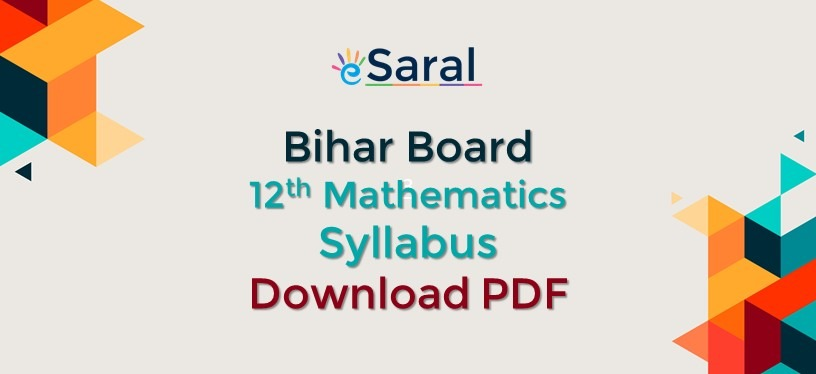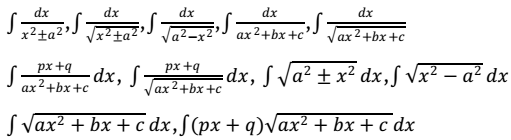Most Affordable JEE | NEET | 8,9,10 Preparation by Kota's Top IITian Doctor Faculties`
Students who are preparing for Bihar Board Class 12 Exam will get here Class 12 Math Syllabus - Bihar Board with the unit-wise Marks distribution. This will help the aspirants to know about the chapters having more weightage. Also get the PDF of Detailed Syllabus in this Article.

Unit-Wise Distribution of Marks
 UNIT NAME Marks UNIT:1 Relation and Function 10 UNIT:2 Calculus 44 UNIT:3 Algebra 13 UNIT:4 Probability 10 UNIT:5 Vector and 3D-Geometry 17 UNIT:6 Linear-Programming Problem 06
Detailed Syllabus for Mathematics Class 12th Unit 1: Relations and Functions
• Relations and Functions: Types of relations: Reflexive, Symmetric, Transitive and Equivalence relations.
• Functions: One to one and onto functions, composite functions, inverse of a function. Binary operations.
• Inverse Trigonometric Functions: Definition, range, domain, principal value branch.
• Graphs of inverse trigonometric functions. Elementary properties of inverse trigonometric functions.
Unit 2: Algebra Matrices
• Basics: Concept, notation, order, equality, types of matrices, zero and identity matrix, transpose of a matrix, symmetric and skew symmetric matrices.
• Operation on matrices: Addition and multiplication and multiplication with a scalar. Simple properties of addition, multiplication and scalar multiplication.
• Non-Commutativity of multiplication of matrices and existence of non-zero matrices whose product is the zero matrix (restrict to square matrices of order
• Concept of elementary row and column operations. Invertible matrices and proof of the uniqueness of inverse, if it exists; (Here all matrices will have real entries).
Determinants
• Determinant of a square matrix (up to 3 x 3 matrices), properties of determinants, minors, cofactors and applications of determinants in finding the area of a triangle.
• Adjoint and inverse of a square matrix.
• Consistency, inconsistency and number of solutions of system of linear equations by examples, solving system of linear equations in two or three variables (having unique solution) using inverse of a matrix.
Unit 3: Calculus Continuity and Differentiability
• Derivative of composite functions, chain rule, derivatives of inverse trigonometric functions, derivative of implicit functions.
• Concept of exponential and logarithmic functions.
• Derivatives of logarithmic and exponential functions. Logarithmic differentiation, derivative of functions expressed in parametric forms. Second order derivatives.
• Rolle’s and Lagrange’s Mean Value Theorems and their geometric interpretation.
Applications of Derivatives
• Rate of change of bodies, increasing/decreasing functions, tangents and normal, use of derivatives in approximation, maxima and minima.
• Simple problems (based on basic principles and understanding of the subject as well as real-life situations).
Integrals
• Integration as the inverse process of differentiation.
• Integration of a variety of functions by substitution, by partial fractions and by parts
• Evaluation of simple integrals of the following types and problems based on them.• Definite integrals as a limit of a sum, Fundamental Theorem of Calculus (without proof). Basic properties of definite integrals and evaluation of definite integrals.
Applications of Integrals
• Applications in finding area under simple curves, Straight lines, circles/parabolas/ellipses.
• Area between any of the two above said curves (the region should be clearly identifiable)
Differential Equations
• Definition, order and degree, general and particular solutions of a differential equation.
• Formation of differential equation whose general solution is given.
• Solution of differential equations by the method of separation of variables solutions of homogeneous differential equations of first order and first degree.
• Solutions of linear differential equation of the type:
• dy/dx + py = q, where p and q are functions of x or constants.
Unit 4: Vectors and 3-Dimensional Geometry Vectors
• Vectors and scalars, magnitude and direction of a vector.
• Direction cosines and direction ratios of a vector.
• Types of vectors, position vector of a point, negative of a vector, components of a vector, addition of vectors, multiplication of a vector by a scalar, position vector of a point dividing a line segment in a given ratio.
• Geometrical Interpretation, properties and application of scalar (dot) product of vectors, vector (cross) product of vectors, scalar triple product of vectors.
3 – Dimensional Geometry
• Direction cosines and direction ratios of a line joining two points.
• Cartesian equation and vector equation of a line, coplanar and skew lines, shortest distance between two lines.Cartesian and vector equation of a plane.
• Distance of a point from a plane.
• Angle between
1. two lines,
2. two planes
3. a line and a plane
Unit 5: Linear Programming
• Introduction
• Related terminology: constraints, objective function, optimization, different types of linear programming (L.P.) problems.
• Mathematical formulation of L.P. problem.
• Graphical method of solution for problems in two variables, feasible and infeasible regions (bounded and unbounded), feasible and infeasible solutions
• Optimal feasible solutions.
Unit 6: Probability
• Multiplication theorem on probability
• Conditional probability
• Independent events, total probability, Baye’s theorem
• Random variable and its probability distribution
• Mean and variance of the random variable
• Repeated independent (Bernoulli) trials & Binomial distribution.
This information would be useful for those who are looking for Mathematics Class 12th Syllabus of Bihar Board. Get to know the detailed syllabus of Class 12th for all Subjects and prepare accordingly for upcoming Exams. Download eSaral App and get access to interactive learning and Video lectures related to Boards, JEE & NEET Preparation. CLICK HERE & Get Bihar Board Syllabus 12th Science 2019 in PDF form.

sanjeevkumarsuman
May 12, 2023, 6:35 a.m.
Join Kar lo
Abahy kumar
Nov. 5, 2022, 9:04 p.m.
nice
Ankita Kumari
Aug. 16, 2023, 5:49 p.m.
Shivay Singh rajput
Aug. 23, 2023, 6:35 a.m.
I love you
Sunny kumar
March 30, 2022, 6:20 p.m.
Sunny kumar
Piyush Priyam
Oct. 8, 2021, 2:38 p.m.